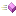The methods of the BitConverter class are listed here.

 Name Descriptionpublic static long toLongFromFourBytes(byte[] bytes, int index) throws Exception { byte[] array = new byte; //System.arraycopy(bytes, 0, array, 4, bytes.length); array = bytes; array = bytes; array = bytes; array = bytes; ByteBuffer b = ByteBuffer.wrap(array);return b.getLong(); } public static long toLongFromUint32(byte[] bytes, int index) throws Exception { byte[] array = new byte; // System.arraycopy(bytes, 0, array, 4, bytes.length); array = (byte) 0; array = (byte) 0; array = (byte) 0; array = (byte) 0; array = bytes[index+0]; array = bytes[index+1]; array = bytes[index+2]; array = bytes[index+3]; // ByteBuffer b = ByteBuffer.wrap(array);// return b.getLong(); long l = ((bytes[index+3]... moreThis is doubleToInt64Bits, a member of class BitConverter.This is getBufferBytes, a member of class BitConverter.This is the overview for the getBytes method overload.This is the overview for the getFourBytes method overload.This is getSixBytes, a member of class BitConverter.This is getTwoBytes, a member of class BitConverter.This is int64BitsToDouble, a member of class BitConverter.This is reverseBytes, a member of class BitConverter.This is the overview for the toBool method overload.This is toBoolean, a member of class BitConverter.This is the overview for the ToBoolean method overload.This is the overview for the ToByte method overload.This is toChar, a member of class BitConverter.This is toDouble, a member of class BitConverter.This is the overview for the ToDouble method overload.public static short toInt16Backwards(byte[] bytes, int index)This is toInt32, a member of class BitConverter.public static int toInt32bb(byte[] bytes, int index) throws Exception { byte[] array = Arrays.copyOfRange(bytes, index, index + 4); ByteBuffer b = ByteBuffer.wrap(array); b.order(ByteOrder.LITTLE_ENDIAN); return b.getInt();This is toInt64, a member of class BitConverter.This is the overview for the toString method overload.This is ToString, a member of class BitConverter.This is toUInt16, a member of class BitConverter.This is ToUInt16, a member of class BitConverter.public static int ToUInt32(byte[] bytes, int index) throws Exception { return toInt32(bytes, index);This is toUInt64, a member of class BitConverter.Change this to ToUInt16